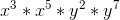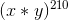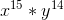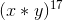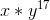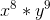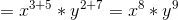Example Questions

Example Question #6 : How To Add Exponents

Simplify: hn + h–2n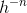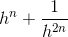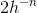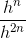Explanation:

h–2n = 1/h2n

hn + h–2n = hn + 1/h2n

Example Question #7 : How To Add Exponents

Simplify: 3y2 + 7y2 + 9y3 – y3 + y

10y2 + 8y3 + y

10y2 + 10y3 + y

10y4 + 8y6 + y

19y11

10y2 + 9y3

10y2 + 8y3 + y

Explanation:

Add the coefficients of similar variables (y, y2, 9y3)

3y2 + 7y2 + 9y3 – y3 + y =

(3 + 7)y2 + (9 – 1)y3 + y =

10y2 + 8y3 + y

Example Question #8 : How To Add Exponents

Simplify the following: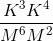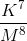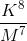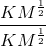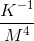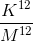Explanation:

When common variables have exponents that are multiplied, their exponents are added. So K* K4 =K(3+4) = K7.  And M6 * M2 = M(6+2) = M8. So the answer is K7/M8.

Example Question #9 : How To Add Exponents

Solve for: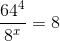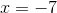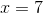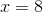Explanation:

First, reduce all values to a common base using properties of exponents.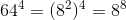Plugging back into the equation-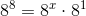Using the formula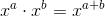We can reduce our equation to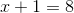So,Example Question #84 : Exponents

Simplify:  y3x4(yx3 + y2x2 + y15 + x22)

y4x7 + y5x6 + y18x4 + y3x26

y3x12 + y6x8 + y45 + x88

2x4y4 + 7y15 + 7x22

y3x12 + y6x8 + y45x4 + y3x88

y3x12 + y12x8 + y24x4 + y3x23

y4x7 + y5x6 + y18x4 + y3x26

Explanation:

When you multiply exponents, you add the common bases:

y4 x7 + y5x6 + y18x4 + y3x26

Example Question #85 : Exponents

If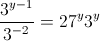, what is the value of?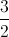Explanation:

Rewrite the term on the left as a product. Remember that negative exponents shift their position in a fraction (denominator to numerator).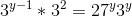The term on the right can be rewritten, as 27 is equal to 3 to the third power.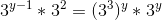Exponent rules dictate that multiplying terms allows us to add their exponents, while one term raised to another allows us to multiply exponents.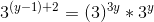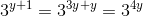We now know that the exponents must be equal, and can solve for.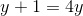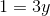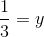Example Question #86 : Exponents

If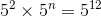, what is the value of?Explanation:

Since the base is 5 for each term, we can say 2 + n =12.  Solve the equation for n by subtracting 2 from both sides to get n = 10.

Example Question #41 : Exponential Operations

Which expression is equivalent to the following?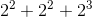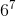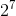None of these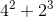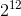None of these

Explanation:

The rule for adding exponents is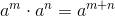. We can thus see that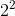and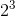are no more compatible for addition thanand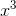are.

You could combine the first two terms into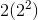, but note that PEMDAS prevents us from equating this to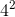(the exponent must solve before the distribution).

Example Question #42 : Exponential Operations

Express as a power of 2: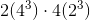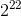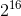The expression cannot be rephrased as a power of 2.Explanation:

Since the problem requires us to finish in a power of 2, it's easiest to begin by reducing all terms to powers of 2. Fortunately, we do not need to use logarithms to do so here.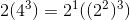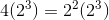Thus,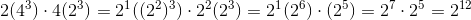Example Question #43 : Exponential Operations

Simplify the following expression: